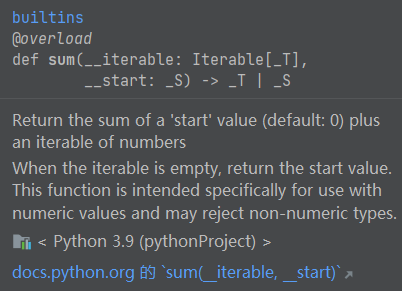• python中sum函数
2020-12-28 21:44:20

python编写一个函数my_sum1，其功能是根据给定的数N求得从1到N间所有数的和并返回

# 包含N

def my_sum1(N):

return sum([x for x in range(1, N 1)])

print my_sum1(10)

python sum函数怎么写

sum是python中很实用的函数，但是要它的使用，我第一次用的时就把它这了：

s

=

sum(1,2,3)

结果就悲剧啦

其实sum()的参数是一个list

例如：

sum([1,2,3])

sum(range(1,11))

还有一个比较有意思的用法

a

=

range(1,11)

b

=

range(1,10)

c

=

sum([item

for

item

in

a

if

item

in

b])

print

c

输出：

45

求问python 2 中的print sum()在python 3 中要如何表达，sum()为函数，谢谢。

搜一下：求问python

2

中的print

sum()在python

3

中要如何表达，sum()为函数，谢谢。

python的sum函数怎么用？

sum(iterable[, start]) ，iterable为可迭代对如：

sum([ ], start)  ， #iterable为list列表。

sum(( ), start ) ， #iterable为tuple元组。

的值=可对应里面的数相加的值 start的值

start默认为0，如果就是0，为0时可以不写，即sum()的参数最多为两个，其中第一个必须为iterable。

版权声明：本站所有文章皆为原创，欢迎转载或转发，请保留网站地址和作者信息。

更多相关内容
• python中sum函数的axis参数取值

格式：sum（a，axis=0）或者是a.sum(axis=1)

sum默认的axis=0 就是普通的相加 ，而当加入axis=1以后就是将一个矩阵的每一行向量相加。

举例：
1.

import numpy as np

np.sum([[0,1,2],[2,1,3]],axis=1)


结果：array（[3,6]）

a = np.array([[0, 2, 1]])

print a.sum()
print a.sum(axis=0)
print a.sum(axis=1)


结果：3, [0, 2, 1], 

b = np.array([0, 2, 1])

print b.sum()
print b.sum(axis=0)
print b.sum(axis=1)


结果：3, 3, 第三个报错，因为和第二个例子不用在于一维数组只有一行，不需要对每一行向量进行求和

c = np.array([[0, 2, 1], [3, 5, 6], [0, 1, 1]])

print c.sum()
print c.sum(axis=0)
print c.sum(axis=1)


结果：19, [3, 8, 8], [3, 14, 2]
这里axis = 0是对应的列进行相加，axis=1是每一行的向量进行相加

展开全文机器学习 算法 numpy
• ## python中sum函数详解

千次阅读 2019-09-11 14:48:38
python内置的sum函数 对象是列表，元组，集合，可以混合起来使用 >>>sum([1,2,3],2) 8 >>>sum((1,2,3)) 6 >>>sum({1,2,3}) 6 >>>sum(range(5)) 10 numpy中sum...

## python3中sum的用法

### python内置的sum函数

对象是列表，元组，集合，可以混合起来使用


>>>sum([1,2,3],2)
8
>>>sum((1,2,3))
6
>>>sum({1,2,3})
6
>>>sum(range(5))
10



### numpy中sum函数


import numpy as np
>>>np.sum([[1,2,3], [4,5,6]])
21
>>>np.sum([[1,2,3],[4,5,6]], axis=0)
[5, 7, 9]
>>>np.sum([[1,2,3],[4,5,6]], axis=1)
[6, 15]



axis的取值有三种情况：1.None，2.整数， 3.整数元组。
（在默认/缺省的情况下，axis取None）
如果axis取None，即将数组/矩阵中的元素全部加起来，得到一个和。
(如果注明了类型，会先将数组中的数字进行类型转换，再求和）

>>> np.sum([0.5, 1.5])
2.0
>>> np.sum([0.5, 0.7, 0.2, 1.5], dtype=np.int32)
1
>>> np.sum([0.5, 0.7, 0.2, 1.5])
2.9
>>> np.sum([[0, 1], [0, 5]])
6


如果axis为整数，axis的取值不可大于数组/矩阵的维度，且axis的不同取值会产生不同的结果。
先以2×2的二维矩阵为例：

>>> np.sum([[0, 1], [0, 5]], axis=0)
array([0, 6])
>>> np.sum([[0, 1], [0, 5]], axis=1)
array([1, 5])
1


在上述例子中
当axis为0时,是压缩行,即将每一列的元素相加,将矩阵压缩为一行
当axis为1时,是压缩列,即将每一行的元素相加,将矩阵压缩为一列
具体理解如图：

当axis取负数的时候，对于二维矩阵，只能取-1和-2（不可超过矩阵的维度）。
当axis=-1时，相当于axis=1的效果，当axis=-2时，相当于axis=0的效果。

如果axis为整数元组（x，y），则是求出axis=x和axis=y情况下得到的和。
继续以上面的2×2矩阵为例

>>>np.sum([[1，2，3],[3，4，5]],axis=(0,1))
>>>6
>>>np.sum([[1，2，3],[3，4，5]],axis=(1,0))
>>>6
>>>np.sum([[[1,2],[3,4]],[[2,3],[4,5]]],axis = (0,1))
>>>[10,14]
>>>np.sum([[[1,2],[3,4]],[[2,3],[4,5]]],axis = (0,1,2))
>>>24


另外，需要注意的是:如果要输入两个数组/矩阵/向量进行相加,那么就要先把两个数组/矩阵/向量用一个括号括起来,形成一个元组,这样才能够进行相加。因为numpy.sum的运算实现本质是通过矩阵内部的运算实现的。

当然,如果只是向量/数组之间做加法运算,可以直接让两个向量/数组相加,但前提是它们必须为numpy的array数组才可以,否则只是单纯的列表相加

>>>v1 = [1, 2]
>>>v2 = [3, 4]
>>>v1 + v2
[1, 2, 3, 4]

>>>v1 = numpy.array[1, 2]
>>>v2 = numpy.array[3, 4]
>>>v1 + v2
[4, 6]

展开全文• 注意：iterable – 可迭代对象...sum=iterable的和+start的值 1、列表 print(sum([1, 2])) print(sum([1, 2], 3)) 3 6 2、元组 print(sum((1, 2))) 3 3、字典（只对键相加） print(sum({1: 3, 2: 3})) 3 ...注意：iterable – 可迭代对象，如：列表(list)、元组(tuple)、字典(dictionary)。

start:如果没写start的值，则默认为0;

sum=iterable的和+start的值

## 1、列表

print(sum([1, 2]))
print(sum([1, 2], 3))
3
6

## 2、元组

print(sum((1, 2)))
3

## 3、字典（只对键相加）

print(sum({1: 3, 2: 3}))
3

## 4、对0:100范围的整数相加

print(sum(range(0, 101)))
5050

### range（a,b）:左闭右开 — a=<val<b

展开全文开发语言 后端
• 今天小编就为大家分享一篇基于Python中求和函数sum的用法详解，具有很好的参考价值，希望对大家有所帮助。一起跟随小编过来看看吧
• sum(iterable[, start]) ，iterable为可迭代对象，如：sum([ ], start) ， #iterable为list列表。sum(( ), start ) ， #iterable为tuple元组。最后的值=可迭代对应里面的数相加的值 + start的值start默认为0，如果不...
• ## Pythonsum()函数

千次阅读 2022-02-20 16:58:22
Python里的sum函数语法例子1. 列表的元素为数字：2. 列表的元素为字符串：3. 列表元素为列表 语法 sum(iterable, start) 参数1 iterable，一个可迭代对象，可以是列表、字典、元祖等，重要的是可迭代对象的...
• #!/usr/bin/env python # -*- coding: utf-8 -*- ...# # sum 函数原型是 sum(iterable, start), 还可以给个初始值 print(sum([1, 2, 3], 10)) # 16 # 另外一个比较Hack一些的用法, 展开2层的嵌套列
• sum()是求和函数，语法如下:sum(iterable[, start])参数解释：iterable -- 可迭代对象，如：列表、元组、集合、字典。(易错点:牢记iterable的元素必须是数值)start -- 指定相加的参数，如果没有设置这个值，默认为...
• sum函数是对系列求和的函数 1.语法： sum(iterable[, start]) 2.参数 iterable -- 可迭代对象，如：列表、元组、集合。 start -- 指定相加的参数，如果没有设置这个值，默认为0。 3.实例 ...
• ## python中sum函数

千次阅读 2017-01-25 17:13:50
python自带的sum函数（或者Numpysum函数），无参时，所有全加；axis=0，按列相加；axis=1，按行相加 import numpy as np #python中自带的sum print(sum([[1,2,3],[4,5,5]])) print(sum([[1,2,3],[4,5,5]],...numpy
• python求和函数sum()的使用示例发布时间：2020-11-21 11:59:04来源：亿速云阅读：59作者：小新小编给大家分享一下python求和函数sum()的使用示例，相信大部分人都还不怎么了解，因此分享这篇文章给大家参考一下，...
• sum(iterable[, start]) ，iterable为可迭代对象，如：sum([ ], start) #iterable为list列表sum(( ), start ) #iterable为tuple元组......最后的值 = 可迭代对象里面的数相加的值 + start的值start默认为0，如果不写...
• 一:python3内置的sum函数用法:sum(iterable, start)iterable:可迭代对象(如列表,元组,集合)start:指定相加的参数,如果没有设置这个值,默认为0返回一个指定相加的参数即stardt(默认为0)加上一个数字的可迭代对象的...
• 拓展：NumPy中sum函数 求相似度时看到的，碰到一个疑问的地方，先写下来。 《机器学习基础：相似度和距离度量究竟是什么》 Python内置的sum函数 作用：对可迭代对象进行求和计算。 sum(iterable[, start]) ...
• ## sum函数语法与使用

千次阅读 2020-11-25 13:40:15
一、sum 函数语法：SELECT SUM(expression ) FROM tables WHERE predicates;expression常量、列或函数，或者是算术、按位与字符串等运算符的任意...二、使用SUM函数需要注意以下几点：（1）含有索引的字段能够加快聚...
• sumpython中一个很实用的函数，但是要注意它的使用，我第一次用的时候，就把它这样用了： 1 s=sum(1,2,3) 结果就悲剧啦 其实sum()的参数是一个list 例如： 1 2 sum(...开发语言 后端
• sum 函数作为 Python 内置函数，顾名思义，可以对迭代器的所有元素求总和，语法如下： ''' 参数介绍： iterable — 可迭代对象，如：列表、元组、集合； start — 指定相加的参数，如果没有设置这个值，默认为0...
• python中sunm函数使用分为两种情况，一种是python自带的sum函数，输入对象是可迭代的。另一种是numpysum函数，对于数组可以指定维度进行相加。以下是这两种情况的具体介绍。一、python自带的sum函数python自带...
• Numpy中sum函数Python自带sum函数）的作用是对元素求和。 无参时，所有全加； axis=0，按列相加； axis=1，按行相加； 下边通过例子来说明其用法： #!/usr/bin/env python # -*- coding:utf-8 -*- from numpy...numpy
• 请阅读以下问题：问题：编写一个函数word_count（string，word），该函数使用列表理解和sum（）函数计算单词在字符串出现的次数。把这个应用到狄更斯弦上。提示：sum（）函数可用于添加列表元素。例如，总和（[1，...
• ## Python中的sum()详解

千次阅读 2021-02-03 10:00:25
sum : sum(iterable, [start=0] ) :求和运算参数说明：iterable：可迭代对象，如列表。start：指定相加的参数，如果没有设置这个值，默认为0list for sum()print(sum([0,1,2])) # 无第二个参数，默认为0 结果=3print...
• ## pythonsum函数怎么用

千次阅读 2021-01-28 19:02:53
之前误用sum()函数，我又去查了查python sum()函数才恍然大悟。我本来想算几个Int值相加的和，本以为很简单的事情，结果却很悲伤，例：>>>sum=sum(1,2,3)#结果很明显出现问题报错TypeError:...
• sum求和函数怎么在Python中使用发布时间：2021-01-12 15:10:47来源：亿速云阅读：55作者：Leah本篇文章为大家展示了sum求和函数怎么在Python中使用，内容简明扼要并且容易理解，绝对能使你眼前一亮，通过这篇文章的...
• 天在学习的过程，误用sum()函数，我又去查了查python sum()函数才恍然大悟。 我本来想算几个Int值相加的和，本以为很简单的事情，结果却很悲伤，例： >>>sum = sum(1,2,3) #结果很明显出现问题报错 ...开发语言
• 今天小编就为大家分享一篇对python中矩阵相加函数sum()的使用详解，具有很好的参考价值，希望对大家有所帮助。一起跟随小编过来看看吧...